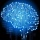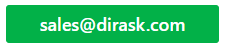Home
IT Knowledge
IT Career
Inspiration
Languages
EN

# Java transpose matrix

3 pointsRoot-ssh
68500

How to transpose matrix in java?

I have:

``````int[][] matrix = {
{1, 2, 3},
{4, 5, 6},
{7, 8, 9}
};``````

And I expect:

``````int[][] transposed = {
{1, 4, 7},
{2, 5, 8},
{3, 6, 9}
};``````

3 pointsRoot-ssh
68500

Quick solution (it works for square and rectangular matrixes) :

``````public static int[][] transposeMatrix(int[][] matrix) {

int[][] temporary = new int[matrix.length][matrix.length];

for (int i = 0; i < matrix.length; i++) {
for (int j = 0; j < matrix.length; j++) {
temporary[j][i] = matrix[i][j];
}
}
return temporary;
}``````

## Example 1

Below example transpose square matrix of size 3 x 3 (3 rows, 3 columns), with total 9 elements. Transposition logic is the same as in quick solution above.

``````import java.util.Arrays;

public class TransposeMatrix {

public static int[][] transposeMatrix(int[][] matrix) {
int[][] temporary = new int[matrix.length][matrix.length];
for (int i = 0; i < matrix.length; i++) {
for (int j = 0; j < matrix.length; j++) {
temporary[j][i] = matrix[i][j];
}
}
return temporary;
}

public static void main(String[] args) {
int[][] matrix = {
{1, 2, 3},
{4, 5, 6},
{7, 8, 9}
};
System.out.println("# matrix before transposition:");
for (int[] row : matrix) {
System.out.println(Arrays.toString(row));
}
int[][] transposed = transposeMatrix(matrix);
System.out.println("# matrix after transposition:");
for (int[] row : transposed) {
System.out.println(Arrays.toString(row));
}
}
}``````

Output:

``````# matrix before transposition:
[1, 2, 3]
[4, 5, 6]
[7, 8, 9]
# matrix after transposition:
[1, 4, 7]
[2, 5, 8]
[3, 6, 9]``````

## Example 2

Below example transpose rectangular matrix of size 2 x 4 (2 rows, 4 columns), with total 8 elements. Transposition logic is the same. We only change the input matrix.

``````import java.util.Arrays;

public class TransposeMatrix2 {

public static int[][] transposeMatrix(int[][] matrix) {
int[][] temporary = new int[matrix.length][matrix.length];
for (int i = 0; i < matrix.length; i++) {
for (int j = 0; j < matrix.length; j++) {
temporary[j][i] = matrix[i][j];
}
}
return temporary;
}

public static void main(String[] args) {
int[][] matrix = {
{1, 2, 3, 4},
{5, 6, 7, 8}
};
System.out.println("# matrix before transposition:");
for (int[] row : matrix) {
System.out.println(Arrays.toString(row));
}
int[][] transposed = transposeMatrix(matrix);
System.out.println("# matrix after transposition:");
for (int[] row : transposed) {
System.out.println(Arrays.toString(row));
}
}
}``````

Output:

``````# matrix before transposition:
[1, 2, 3, 4]
[5, 6, 7, 8]
# matrix after transposition:
[1, 5]
[2, 6]
[3, 7]
[4, 8]``````

## Some details

In math, transpose of matrix is the process of chaning rows to columns and columns to rows. The process of transposition is quite easy, we just change A[i][j] to A[j][i].
We can do it in couple of different ways.
It is very oftne used in linear algebra.